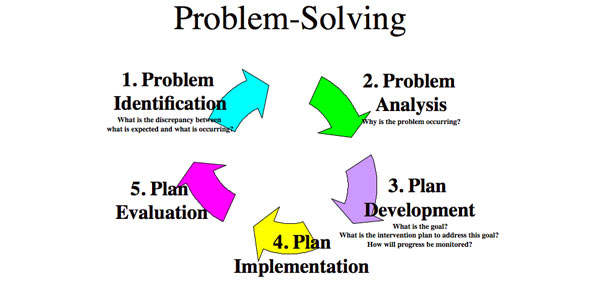# Engineering Design And Problem Solving

Approved & Edited by ProProfs Editorial Team
At ProProfs Quizzes, our dedicated in-house team of experts takes pride in their work. With a sharp eye for detail, they meticulously review each quiz. This ensures that every quiz, taken by over 100 million users, meets our standards of accuracy, clarity, and engagement.
| Written by Dproane
D
Dproane
Community Contributor
Quizzes Created: 4 | Total Attempts: 5,576
Questions: 10 | Attempts: 400SettingsEngineering design and problem solving is a multifaceted topic making use of different engineering, mathematical and graphics models and their uses. Do you want to know more? Take up this quiz and learn more. All the best.

• 1.

### Which of the following is NOT part of the Analytic Problem Solving method:

• A.

Apply theory and equations

• B.

Simplify the problems

• C.

Verify accuracy to the required level

• D.

Diagram and describe

B. Simplify the problems
Explanation
The Analytic Problem Solving method involves applying theory and equations, verifying accuracy to the required level, and diagramming and describing the problem. However, it does not involve simplifying the problems.

Rate this question:

• 2.

### Which of the following is NOT a type of engineering model:

• A.

Physical

• B.

Mathematical

• C.

Functional

• D.

Graphic

C. Functional
Explanation
A functional model is a type of engineering model that represents the behavior and functionality of a system or process. It focuses on how the system works and how its components interact with each other. Physical models represent the physical aspects of a system, mathematical models use mathematical equations to describe and analyze the system, and graphic models use visual representations to communicate information about the system. Therefore, functional is not a type of engineering model as it does not represent the physical or mathematical aspects of a system.

Rate this question:

• 3.

### Which of the following is a mathematical model?

• A.

Work = force x distance

• B.

Energy = mass x speed of light x speed of light

• C.

Force = mass x acceleration

• D.

All of the above

D. All of the above
Explanation
All of the given equations are mathematical models because they represent mathematical relationships between different variables. The equation "work = force x distance" represents the relationship between work, force, and distance. The equation "energy = mass x speed of light x speed of light" represents the relationship between energy, mass, and the speed of light. The equation "force = mass x acceleration" represents the relationship between force, mass, and acceleration. Therefore, all of the given equations can be considered mathematical models.

Rate this question:

• 4.

### Which of the following is NOT a graphic model?

• A.

Prototypes

• B.

Conceptual models

• C.

Graphs

• D.

Diagrams

A. Prototypes
Explanation
Prototypes are not a graphic model because they are physical or digital representations of a product or system, used for testing and evaluation purposes. Graphic models, on the other hand, are visual representations that depict relationships, structures, or concepts. Conceptual models, graphs, and diagrams are all examples of graphic models as they visually represent abstract ideas, data, or relationships.

Rate this question:

• 5.

### Where E = potential, I = current, and R = resistance, Ohm's Law can be expressed as E = V / R.

• A.

True

• B.

False

B. False
Explanation
Ohm's Law states that the potential difference (E) across a conductor is directly proportional to the current (I) flowing through it and inversely proportional to the resistance (R) of the conductor. The correct expression for Ohm's Law is E = I * R, not E = V / R. Therefore, the given answer is false.

Rate this question:

• 6.

### Electrical power is measured in watts.

• A.

True

• B.

False

A. True
Explanation
Electrical power is indeed measured in watts. Watts is the unit used to quantify the rate at which electrical energy is transferred or consumed. It is a measure of the amount of work done or energy used per unit of time. Therefore, the statement "Electrical power is measured in watts" is correct.

Rate this question:

• 7.

### A milliamp = 0.01 amps

• A.

True

• B.

False

B. False
Explanation
The statement "A milliamp = 0.01 amps" is incorrect. In reality, a milliamp (mA) is equal to 0.001 amps (A), not 0.01 amps. The prefix "milli-" denotes a factor of 0.001, so a milliamp is one thousandth of an amp. Therefore, the correct answer is False.

Rate this question:

• 8.

### Electrical continuity is a state of an electrical circuit being completely connected and able to conduct current.

• A.

True

• B.

False

A. True
Explanation
Electrical continuity refers to the condition of an electrical circuit where all components are properly connected and can effectively allow the flow of electric current. This means that there are no breaks or interruptions in the circuit that would prevent the current from flowing. Therefore, the statement "Electrical continuity is a state of an electrical circuit being completely connected and able to conduct current" is true.

Rate this question:

• 9.

### A prototype is a physical model showing people how a product or structure will look.

• A.

True

• B.

FalseBack to top